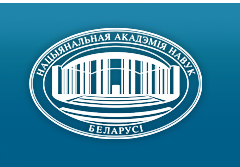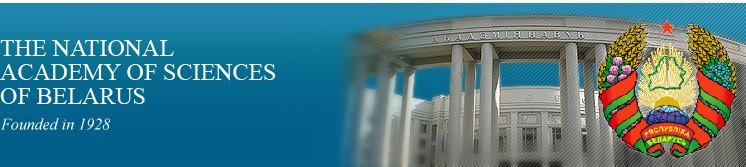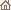Home News About Academy Academy Activities Academy Membership Organizations Publications Conferences Contacts Site Map

# Nonlinear Phenomena in Complex Systems, 1999, Vol.2, No.2/ Publications / Scientific Journals
Nonlinear Phenomena in Complex Systems, 1999, Vol.2, No.2NONLINEAR PHENOMENA IN COMPLEX SYSTEMS An Interdisciplinary Journal Published by The "Education and Upbringing" Publishing Company, Minsk, Republic of Belarus

### Volume 2, Number 2, 1999## CONTENTS

Srinivas Jammalamadaka, Jцrg Main, Gьnter Wunner
The Effect of Scars on the Statistics of Transition Probabilities of Classically Chaotic Quantum Systems. pp. 1--5

Summary: We study the statistical properties of generalized intensities (squared matrix elements of Hermitian operators) for the hydrogen atom in strong magnetic fields in a range of parameters where the classical analogue of the system exhibits completely chaotic dynamics. In this way we extend previous work by Prosen and Robnik on the statistics of generalized intensities in billiard systems in the transition region with mixed classical dynamics. We observe deviations from the statistics found in that work, and demonstrate that these are due to the effect of scarring of wave functions by unstable periodic orbits.

V.P. Gribkovskii, S.V. Voitikov, M.I. Kramar, G.I. Ryabtsev, and R. Kragler
Amplified Luminescence as a Source of Nonlinearity in Laser Diodes. pp. 6--10

Summary: Nonlinear properties of amplified luminescence in semiconductor bulk and quantum-well (QW) lasers have been investigated and modeled. Amplified luminescence depends strictly on carrier concentration and temperature representing by itself an additional relatively powerful source of nonlinearity that can make laser dynamics much more complicated. In general, the rate of amplified luminescence still keeps its quadratic character as that of spontaneous recombination. In QW lasers, in the vicinities of laser mode hopping the amplified luminescence exhibits local peak-like carrier dependence and may affects abruptly on a bifurcation of mode hopping with the injection level increasing.

H.V. Grushevskaya
Chaos in Near-Hamiltonian Systems with Singular Perturbation: Applications to Oscillatory Model of Hodgkin--Huxley Neuron. pp. 11--24

Summary: Neuron's oscillatory model is transformed to the form of near-Hamiltonian system with singular coefficients. We demonstratethat singular coefficients can be considered as a perturbation of the system by virtue of discrete sequence of kicks. The linearization of the perturbation in the vicinity of periodical solution for a given kick allows us to rewrite the system as a matrix Schrцdinger equation for two-level quantum system in a resonant quasi-monochromatic field. As a result, the original system demonstrates chaotic behavior through the cascade of period doubling bifurcations. The bifurcations in this case correspond to the series of quantum nutation of nutations.

A.V. Kartynnick, A.D. Linkevich
Global Attractors, Attracting Regions and Regions of Visiting for Dynamical Systems. pp. 25--30

Summary: The phase space of an autonomous dissipative nonlinear dynamical system is investigated. It is proved that under certain conditions such a system has an attracting region A with a basin of attraction around it, i.e. any trajectory starting somewhere inside this basin reaches the region A after a finite time interval and never leaves A. In certain cases, the region A turns into a global attractor of the system when the basin of attraction coincides with the whole remaining part of the phase space and all attractors of the system lie inside the region A. Another theorem yields sufficient conditions under which a system has a region of visiting G in the phase space, i.e. any trajectory starting outside G reaches this region after a finite time interval (and further can leave G in contrast to the case of an attracting region). Several examples are considered.

V.A. Gaisyonok, G.G. Krylov
On the Green Function for the Restricted Rotational Diffusion Model. pp. 31--34

Summary: The explicit expression for the Green function of the restricted rotational diffusion equation has been constructed based on singular perturbations approach to boundary problems.

Alexander Rauh
Remarks on Perturbation Theory for Hamiltonian Systems. pp. 35--43

Summary: A comparative discussion of the normal form and action angle variable method is presented in a tutorial way. Normal forms are introduced by Lie series which avoid mixed variable canonical transformations. The main interest is focused on establishing a third integral of motion for the transformed Hamiltonian truncated at finite order of the perturbation parameter. In particular, for the case of the action angle variable scheme, the proper canonical transformations are worked out which reveal the third integral in consistency with the normal form. Details are discussed exemplarily for the Hйnon--Heiles Hamiltonian. The main conclusions are generalized to the case of n perturbed harmonic oscillators.

H. Rehfeld, H. Alt, C. Dembowski, H.-D. Grдf, R. Hofferbert, H. Lengeler, A. Richter
Wave Dynamical Chaos in Superconducting Microwave Billiards. pp. 44--48

Summary: During the last few years we have studied the chaotic behavior of special formed Euclidian geometries, so-called billiards, from the quantum or in more general sense "wave dynamical'' point of view. Due to the equivalence between the stationary Schrцodinger equation and the classical Helmholtz equation in the two-dimensional case (plain billiards), it is possible to simulate "quantum chaos'' with the help of macroscopic, superconducting microwave cavities. Using this technique we investigated spectra of three billiards of the family of Pascal's Snails (Robnik--Billiards) with a different chaoticity in each case in order to test predictions of standard stochastical models for classical chaotic systems.

Marko Robnik, Luca Salasnich and Marko Vranicar
WKB Corrections to the Energy Splitting in Double Well Potentials. pp. 49--62

Summary: By using the WKB quantization we deduce an analytical formula for the energy splitting in a double-well potential which is the usual Landau formula with additional quantum corrections. Then we analyze the accuracy of our formula for the double square well potential, the inverted harmonic oscillator and the quartic potential.

Vassilios M. Rothos and Tassos C. Bountis
Non-Integrability and Infinite Branching of Solutions of 2DOF Hamiltonian Systems in Complex Plane of Time. pp.63--71

Summary: It has been proved by S.L. Ziglin, for a large class of 2-degree-of-freedom (d.o.f) Hamiltonian systems, that transverse intersections of the invariant manifolds of saddle fixed points imply infinite branching of solutions in the complex time plane and the non-existence of a second analytic integral of the motion. Here, we review in detail our recent results, following a similar approach to show the existence of infinitely-sheeted solutions for 2 d.o.f. Hamiltonians which exhibit, upon perturbation, subharmonic bifurcations of resonant tori around an elliptic fixed point. Moreover, as shown recently, these Hamiltonian systems are non--integrable if their resonant tori form a dense set. These results can be extended to the case where the periodic perturbation is not Hamiltonian.

Aneta Stefanovska, Saso Strle, Maja Bracic and Hermann Haken
Model Synthesis of the Coupled Oscillators Which Regulate Human Blood Flow Dynamics. pp.72--87

Summary: A model synthesis for the system that regulates blood flow is presented. The model consists of coupled oscillators which present subsystems involved in the regulation of one passage of blood through the cardiovascular system. It is based on a priori physiological knowledge and observations of the system's functions by measurements and linear and nonlinear analysis of measured time series. Furthermore, the blood flow through the system of closed tubes consisting of blood vessels is described by wave equations.

V.V. Belov, G.S. Bokun, V.S. Vikhrenko
Many-body Correlations in Equilibrium Lattice Systems. pp. 88--92

Summary: The procedure of successive approximations beyond the quasichemical one for lattice gas systems of arbitrary density is suggested. Even at the lowest order approximation the calculation results for the phase diagram of the system of particles with attractive nearest neighbor interaction are in good agreement with Monte Carlo simulation.

E.A. Nikiforov and R.M. Yulmetyev
First Experimental Observation of Memory Effects in Biological Complex System by NMR Method. pp. 93--95

Summary: Direct registration of memory effects in biological complex system (plant tissue) has been carried out for the first time. Measurements of temperature dependence of the proton spin-lattice relaxation time in rotation frame allow to observe quasi-Markovian scenario of NMR spin relaxation in biological system.

Sergey Sadov
Lissajous Solutions of the Satellite Oscillation Equation: Stability and Bifurcations via Higher Order Averaging. pp.96--100

Summary: The equation of plane oscillations of a satellite is a Hamiltonian system with one degree of freedom and 2p-periodic dependence on time and on x1. It contains two parameters $e\eps$ and e, one of which ($\eps$) is small. The unperturbed system is linear. Solutions that correspond to cycles of the Poincar\'e map on a cylinder $(x1\mpi,\,x2)$ are called Lissajous solutions. Their stability and bifurcations with parameter e changing are studied by the averaging method. It is shown how degenerate cases, where calculation of higher order terms is needed, arise in a natural way. Sufficient truncations of the normal form for those cases are described.

Andreas Ruffing
Discretized Schroedinger Eigenfunctions and q-Hypergeometric Series on Deforming Geometric Progressions. pp.101--116

Summary: Discretizations of the Schroedinger equation are introduced on geometric progressions $\R_{q} := \{+q^{n}, -q^{n} \vert n \in \Z\}, q > 1$. The symmetries of the geometric progressions are elaborated. We investigate the influence of this discretization to q-deformations of Hermite polynomials. The limits $q \rightarrow 1$ and $q \rightarrow \infty$ as deformations of $\R_{q}$ are considered. The first limit, $q \rightarrow 1$, is related to an approximation theoretical problem for step functions in ${\cal{L}}^{2}(\R)$. The second limit, $q \rightarrow \infty$, is related to the theory of topological deformations on compact Riemann surfaces. Both limits are related to each other. Proceeding into this direction, one obtains the fascinating fact that quantum group structures can be related to topological degeneration effects. The results finally contribute to a better mathema-tical understanding of quantum models with dilatative supersymmetry.

Designed and maintained by Dr. Nikolai N. Kostyukovich. Last updated: October 31, 1999
Created with assistance of Dr. Leonid F. Babichev
Copyright © 1999 The National Academy of Sciences of Belarus
Copyright © 1999 The Nonlinear Phenomena in Complex Systems